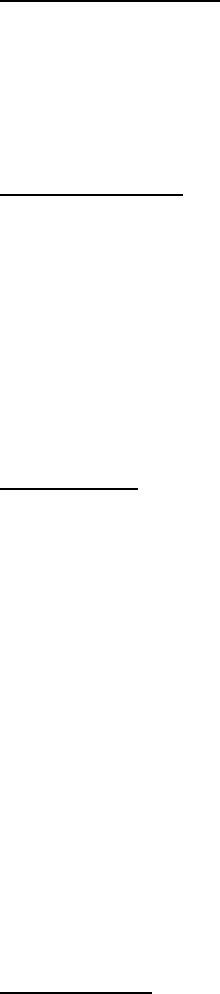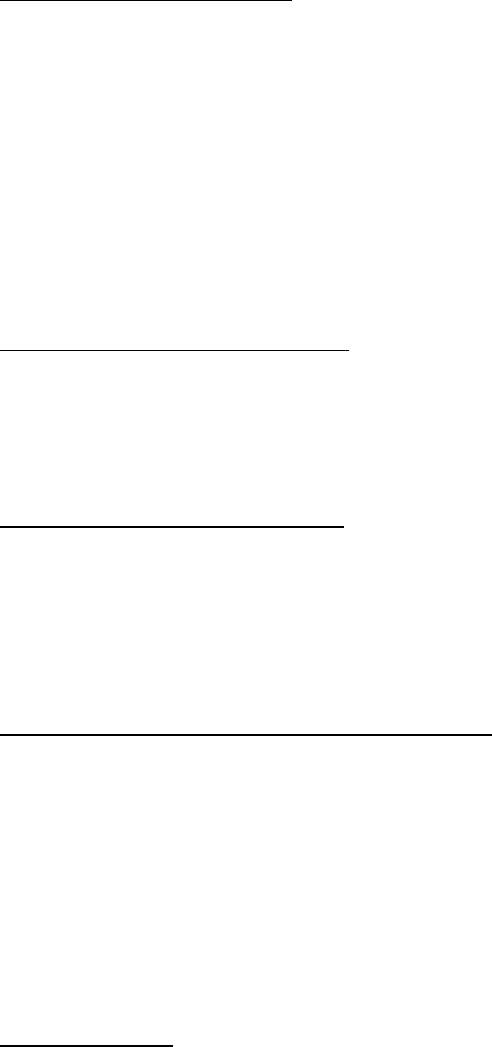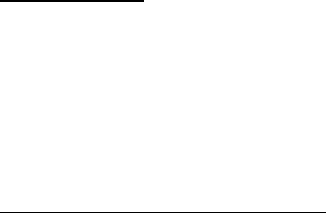# COMMERCE 3QC3 Chapter 12&15: 2OC3 - Chapter 12&15.docx

30 views8 pagesCHAPTER TWELVE: INVENTORY MANAGEMENT
The Importance of Inventory
Objective of inventory management is to strike a balance between inventory
investment and customer service
Can never achieve low-cost strategy without good inventory management
Functions of Inventory
1. To separate various parts of production process
2. To separate firm from fluctuations in demand and provide stock of goods that will
provide selection to customers
3. To take advantage of quantity discounts
4. To hedge against inflation (and upward price changes)
Types of Inventory
Raw material inventory: materials purchased but have yet to enter manufacturing
process
Work-in-process (WIP) inventory: products that are not raw materials but not
finished products
WIP exists because of time it takes for product to be made (cycle time)
MRO: inventories devoted to maintenance/repair/operating supplies
Finished-goods inventory: end item ready to be sold
Managing Inventory
ABC Analysis
Divides on-hand inventory into three classifications on the basis of annual dollar
volume
Idea is to establish inventory policies that focus resources on the few critical
inventory parts and not the trivial ones
Should not monitor inexpensive items with the same intensity as very expensive
items
Class A items:
oAnnual dollar volume is high
oMay represent only 15% of inventory but 70-80% of dollar usage
Class B items:
oMedium annual dollar volume
oRepresent 30% of inventory items and 15-25% of total value
Class C items:
oLow annual dollar volume
o55% of inventory items but 5% of annual dollar volume
Cycle Counting
Inventory records must be verified through a continuing audit
Cycle counting: continuing reconciliation of inventory with inventory records
Unlock document

This preview shows pages 1-3 of the document.
Unlock all 8 pages and 3 million more documents.Items are counted, records are verified and inaccuracies are periodically
documented
A items counted once a month
B items counted once a quarter
C items counted once every 6 months
Control of Service Inventories
Shrinkage: retail inventory that is unaccounted for between receipt and sale
Occurs from damage and theft
Pilferage: small amount of inventory theft
Techniques for ensuring inventory accuracy and control:
1. Good personnel selection, raining and discipline
2. Tight control of incoming shipments
3. Effective control of all goods leaving the facility
Major retailers lose 10% to 25% of overall profits due to poor or inaccurate
inventory records
Inventory Models
Independent vs. Dependent Demand
Assume that demand for an item is either independent of or dependent on the
demand for other items
Demand for fridges is independent of demand for toasters
Demand for toaster oven components is dependent on demand for toaster ovens
Holding, Ordering, and Setup Costs
Holding costs: costs to keep or carry inventory in stock
Ordering cost: cost of ordering process
Setup cost: cost to prepare a machine for manufacturing an order
Setup time: time required to prepare a machine or process for production
Inventory Models for Independent Demand
The Basic Economic Order Quantity (EOQ) Model
Minimizes total of ordering and holding costs
Based on several assumptions:
1. Demand for item is known, constant and independent of other items
2. Lead time is known and consistent
3. Receipt of inventory is instantaneous and complete
4. Quantity discounts are not possible
5. Only variable costs are cost of setting up or placing an order and cost of
holding inventory
6. Shortages can be completely avoided if orders placed at right time
Minimizing Costs
If minimize sum of setup and holding costs we will also be minimizing total costs
Unlock document

This preview shows pages 1-3 of the document.
Unlock all 8 pages and 3 million more documents.As quantity ordered increases the annual setup or ordering cost will decrease
As order quantity increases the holding cost will increase
Optimal order quantity occurs where ordering-cost curve and carrying-cost curve
intersect
Optimal order quantity will occur at point where total setup cost is equal to total
holding cost
Steps for EOQ:
1. Annual setup cost
= (Number of orders placed per year) x (Setup cost per order)
= (Annual demand/Number of units in each order) x (Setup cost per order)
= (D/Q) x (S)
2. Annual holding cost
= (Average inventory level) x (Holding cost per unit per year)
= (Order quantity/2) x (Holding cost per unit per year)
= (Q/2) x (H)
3. Optimal order quantity is found where: (D/Q)(S) = (Q/2)(H)
4. Solve for Q*: Q* = (2DS/H)
Expected number of orders placed per year (N)
= Demand/Order quantity = D/Q*
Expected time between orders (T)
= Number of working days per year/N
Total annual cost (TC) = Setup cost + Holding cost = (D/S)(S) + (Q/2)(H)
If include purchase cost: TC = (D/S)(H) + (Q/2)(H) + PD
Reorder Points
Lead time: time between placement and receipt of an order
Reorder point (ROP): inventory level at which an order should be placed
ROP = (Demand per day) x (Lead time for a new order in days) = d x L
Safety stock: extra stock to allow for uneven demand (a buffer)
Demand per day = d = (Annual demand)/(Number of working days in a year)
Production Order Quantity Model
Firm may receive its inventory over a period of time
This can happen in two situations:
1) When inventory continuously flows or builds up over time after order has
been placed
2) When units are produced and sold simultaneously
Production order quantity model: economic order quantity technique applied to
production orders
Annual inventory holding cost
= (Annual inventory level) x (Holding cost per unit per year)
Unlock document

This preview shows pages 1-3 of the document.
Unlock all 8 pages and 3 million more documents.

# Get access

\$10 USD/m
Billed \$120 USD annually
Homework Help
Class Notes
Textbook Notes